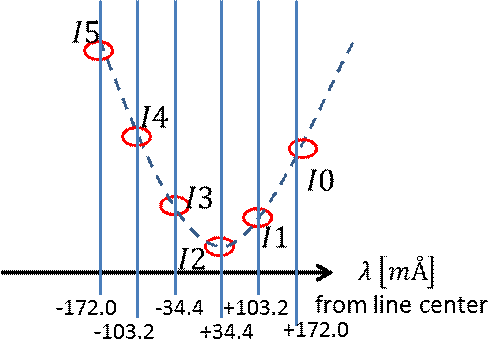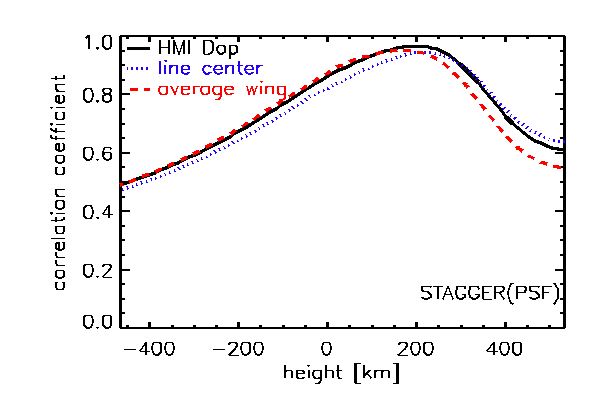# 26. SDO/HMI Multi-height Dopplergrams

Contributed by Kaori Nagashima. Posted on August 15, 2014

Kaori Nagashima1, Björn Löptien2, Laurent Gizon1,2, Aaron C. Birch1, Robert Cameron1, Sebastien Couvidat3, Sanja Danilovic1, Bernhard Fleck4, Robert Stein5

1 Max-Planck-Institut für Sonnensystemforschung, Justus-von-Liebig-Weg 3, 37077 Göttingen, Germany
2 Institut für Astrophysik, Georg-August-Universität Göttingen, 37077 Göttingen, Germany
3 Stanford University, Stanford, CA 94305, USA
4 ESA Science Operations Department, c/o NASA/GSFC, Greenbelt, MD 20771, USA
5 Michigan State University, East Lansing, MI 48824, USA

Both numerical simulations and observations indicate that the plasma velocity varies with height within the solar photosphere. Mapping the velocity simultaneously at several different heights would give us insight into, for example, how waves are affected by radiative transfer above the surface. Multi-height Doppler velocity maps are also important for many other types of studies, e.g. to learn about how energy is transported in the atmosphere.

Obtaining multi-height velocity information from HMI would be advantageous, providing nearly continuous temporal coverage of the entire solar disk at a 45 second cadence and reasonably high spatial resolution. We recently showed that this information can be obtained from the SDO/HMI data.Figure 1 |Conceptual illustration of the Fe I 617.3 nm spectral line and HMI filter positions.

SDO/HMI observes the Sun in the Fe I absorption line at 617.3 nm and takes filtergrams at 6 wavelengths across the line. The standard HMI-algorithm Dopplergram is derived from these 6-wavelength filtergrams . In our recent study , using the same filtergrams, we create two other Dopplergrams: one is the average-wing Dopplergram to see deeper in the photosphere and the other one is the Line-center Dopplergram which responds to velocities higher in the photosphere. This approach is based on the fact that the line center is formed deeper in the atmosphere than the wings of the line.

We calculate the average-wing Dopplergram using (I_b – I_r)/(I_b+I_r), where I_b and I_r are blue- and red-wing averaged intensity, i.e., I_b= (I5+I4)/2 and I_r = (I0+I1)/2 (See Figure 1). The line-center Dopplergrams are derived as the shift of the apex of a parabola through the three points around the minimum intensity wavelength at each horizontal pixel and each temporal step.Figure 2 |Correlation coefficients of the synthetic Doppler velocities and the original vertical velocity field in the simulation box of the STAGGER data. Black-solid: standard HMI-algorithm Dopplergram. Blue-dotted: line-center Dopplergram. Red-dashed: average wing Dopplergram.

To estimate the heights in the atmosphere where these Dopplergrams are formed, we used realistic 3D convection simulations provided by the STAGGER code . We synthesized HMI filtergrams using the SPINOR code  and a model for the HMI point spread function (PSF). We used these synthetic observations to construct the different types of Dopplergrams, which were then compared to the actual vertical velocity field in the simulation box at various heights. Figure 2 shows the correlation coefficients between the synthetic Doppler velocities and the velocity in the simulation box of the STAGGER data. The heights of the peak correlation are 221 km (line center), 195 km (standard HMI-algorithm), and 170 km (average wing). (See  for details.)

Thus, with these two new Dopplergrams obtained from HMI filtergrams, we can obtain velocity information from two layers separated by 50 km.

### References

 Nagashima, K., Löptien, B., Gizon, L., Birch, A. C., Cameron, R., Couvidat, S., Danilovic, S., Fleck B., Stein, R. 2014, SoPh, 289, 3457
 Couvidat, S., Rajaguru, S.P., Wachter, R., Sankarasubramanian, K., Schou, J., Scherrer, P.H. 2012, SoPh, 278, 217
 Stein, R.F. 2012. LRSP, 9 (4)
 Frutiger, C., Solanki, S.K., Fligge, M., Bruls, J.H.M.J. 2000, A&A, 358, 1109